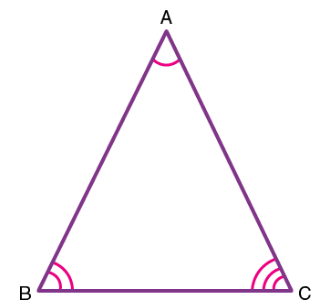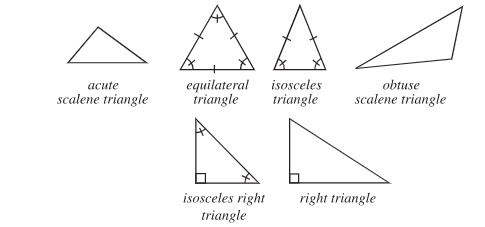Angle sum property of triangle

## Objective

Angle sum property of a triangle states that the sum of interior angles of a triangle is 180°.
i.e. ∠A+∠B+∠C= 180°## Theory

### Triangle

A triangle is a 3-sided polygon sometimes (but not very commonly) called the trigon. Every triangle has three sides and three angles, some of which may be the same. The sides of a triangle are given special names in the case of a right triangle, with the side opposite the right angle being termed the hypotenuse and the other two sides being known as the legs. In Geometry, a triangle is a three-sided polygon that consists of three edges and three vertices. The most important property of a triangle is that the sum of the internal angles of a triangle is equal to 180 degrees. This property is called the angle sum property of a triangle.

### Types of triangle### Angle sum property

The angle sum property of a triangle states that the sum of the angles of a triangle is equal to 180º. A triangle has three sides and three angles, one at each vertex. Whether a triangle is an acute, obtuse, or a right triangle, the sum of its interior angles is always 180º.

The angle sum property of a triangle is one of the most frequently used properties in geometry. This property is mostly used to calculate unknown angles.

## Pre-requisite Knowledge

• Concept of triangles.
• Concept of interior and exterior angles of a triangle
• Concept of straight angles,exterior angle, opposite angle and alternate interior angles
• Area of triangle

The half of the product of its base and height.

Formula : Area = 1/2*b*h